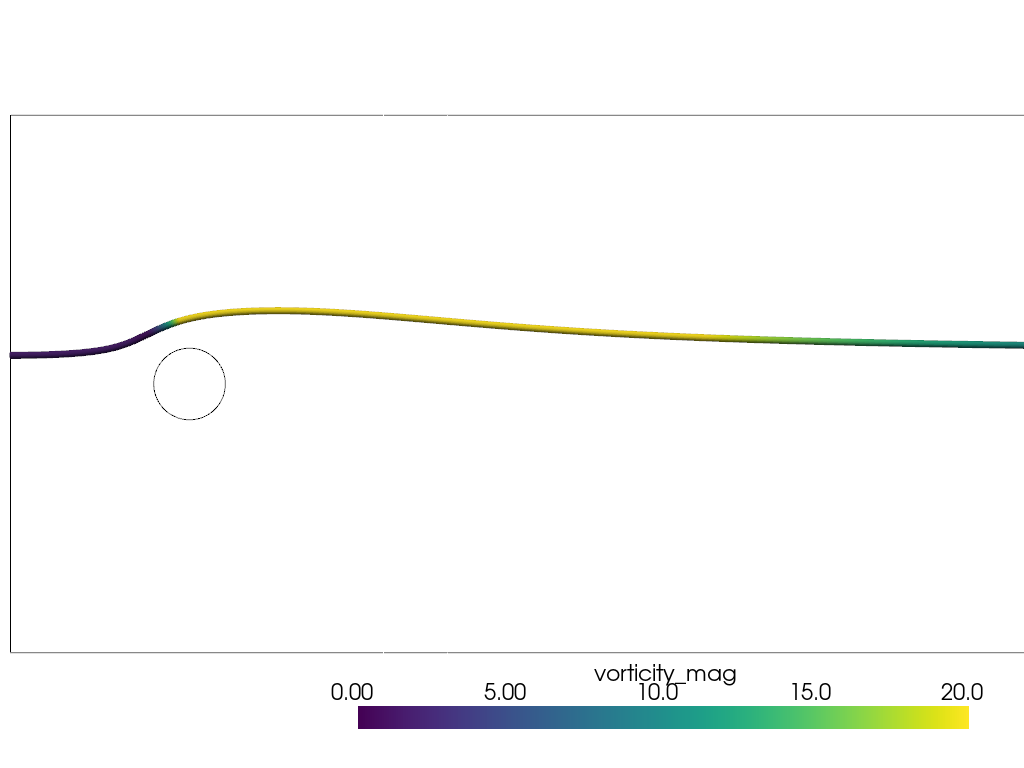# 2D Streamlines#

Integrate a vector field to generate streamlines on a 2D surface.

```
```

This example generates streamlines of flow around a cylinder in cross flow.

```import pyvista as pv
from pyvista import examples
```

The data is multiblock with the fluid data as the first block. The data lies in the xy plane, i.e. z=0, with no z velocity.

```mesh = examples.download_cylinder_crossflow()
fluid_mesh = mesh
print(fluid_mesh)
```

Out:

```UnstructuredGrid (0x7fea0179da00)
N Cells:      14594
N Points:     14831
X Bounds:     0.000e+00, 1.500e+01
Y Bounds:     -3.750e+00, 3.750e+00
Z Bounds:     0.000e+00, 0.000e+00
N Arrays:     3
```

The default behavior of the `streamlines()` filter is to use a 3D sphere source as the seed points. This often will not generate any seed points on the 2D plane of interest. Instead, a single streamline can be generated using the `start_position` argument. The `surface_streamlines=True` argument is also needed if the dataset has nonzero normal velocity component. This is not the case in this dataset.

```one_streamline = fluid_mesh.streamlines(
start_position=(0.0, 0.4, 0.0),
max_time=100.0,
compute_vorticity=False,  # vorticity already exists in dataset
)

clim = [0, 20]
camera_position = [(7, 0, 20.0), (7, 0.0, 0.0), (0.0, 1.0, 0.0)]

p = pv.Plotter()
for i in range(1, len(mesh)):
p.view_xy()
p.show(cpos=camera_position)
```To generate multiple streamlines, a line source can be used with the `pointa` and `pointb` parameters.

```line_streamlines = fluid_mesh.streamlines(
pointa=(0, -5, 0),
pointb=(0, 5, 0),
n_points=25,
max_time=100.0,
compute_vorticity=False,  # vorticity already exists in dataset
)

p = pv.Plotter()
for i in range(1, len(mesh)):
p.view_xy()
p.show(cpos=camera_position)
```The behavior immediately downstream of the cylinder is still not apparent using streamlines at the inlet.

Another method is to use `streamlines_evenly_spaced_2D()`. This filter only works with 2D data that lies on the xy plane. This method can quickly run of memory, so particular attention must be paid to the input parameters. The defaults are in cell length units.

```line_streamlines = fluid_mesh.streamlines_evenly_spaced_2D(
start_position=(4, 0.1, 0.0),
separating_distance=3,
separating_distance_ratio=0.2,
compute_vorticity=False,  # vorticity already exists in dataset
)

p = pv.Plotter()
for i in range(1, len(mesh)):The streamlines are only approximately evenly spaced and capture the vortex pair downstream of the cylinder with appropriate choice of `start_position`.